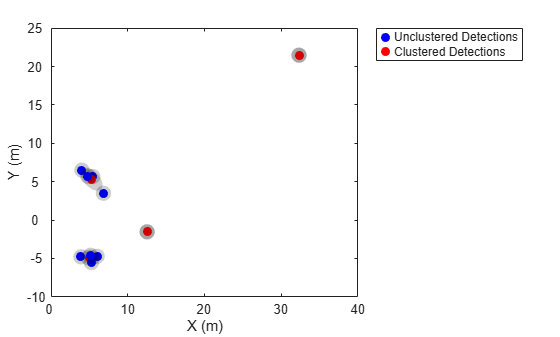# mergeDetections

Merge detections into clustered detections

Since R2021b

## Syntax

``clusteredDetections = mergeDetections(detections,clusterIndex)``
``clusteredDetections = mergeDetections(___,MergingFcn=mergeFcn)``

## Description

example

````clusteredDetections = mergeDetections(detections,clusterIndex)` merges detections sharing the same cluster labels. By default, the function merges detections in the same cluster using a Gaussian mixture merging algorithm. The function assumes that all detections in the same cluster share the same `Time`, `SensorIndex`, `ObjectClassID`, `MeasurementParameters`, and `ObjectAttributes` properties or fields. ```
````clusteredDetections = mergeDetections(___,MergingFcn=mergeFcn)` specifies the function used to merge the detections in addition to the input arguments from the previous syntax.```

## Examples

collapse all

Generate two clusters of detections with two false alarms.

```rng(2021) % For repeatable results x1 = [5; 5; 0] + randn(3,4); % Four detections in cluster one x2 = [5; -5; 0] + randn(3,4); % Four detections in cluster two xFalse = 30*randn(3,2); % Two false alarms x = [x1 x2 xFalse];```

Format these detections into a cell array of `objectDetection` objects.

```detections = repmat({objectDetection(0,[0; 0; 0])},10,1); for i = 1:10 detections{i}.Measurement = x(:,i); end```

Define the cluster indices according to the previously defined scenario. You can typically obtain the cluster indices by applying a clustering algorithm on the detections.

`clusterIndex = [1; 1; 1; 1; 2; 2; 2; 2; 3; 4];`

Use the `mergeDetections` function to merge the detections.

`clusteredDetections = mergeDetections(detections,clusterIndex);`

Visualize the results in a theater plot.

```% Create a theaterPlot object. tp = theaterPlot; % Create two detection plotters, one for unclustered detections and one for % clustered detections. detPlotterUn = detectionPlotter(tp,DisplayName="Unclustered Detections", ... MarkerFaceColor="b",MarkerEdgeColor="b"); detPlotterC = detectionPlotter(tp,DisplayName="Clustered Detections", ... MarkerFaceColor="r",MarkerEdgeColor="r"); % Concatenate measurements and covariances for unclustered detections detArray = [detections{:}]; xUn = horzcat(detArray.Measurement)'; PUn = cat(3,detArray.MeasurementNoise); % Concatenate measurements and covariance for clustered detections clusteredDetArray = [clusteredDetections{:}]; xC = horzcat(clusteredDetArray.Measurement)'; PC = cat(3,clusteredDetArray.MeasurementNoise); % Plot all unclustered and clustered detections plotDetection(detPlotterUn,xUn,PUn); plotDetection(detPlotterC,xC,PC);```## Input Arguments

collapse all

Object detections, specified as an N-element array of `objectDetection` objects, N-element cell array of `objectDetection` objects, or an N-element array of structures whose field names are the same as the property names of the `objectDetection` object. N is the number of detections. You can create `detections` directly, or you can obtain `detections` from the outputs of sensor objects such as `fusionRadarSensor`, `irSensor`, and `sonarSensor`.

Cluster indices, specified as an N-element vector of positive integers, where N is the number of detections specified in the `detections` input. Each element is the cluster index of the corresponding detection in the `detections` input. For example, if `clusterIndex(i)=k`, then the `i`th detection from the `detections` input belongs to cluster `k`.

Function to merge detections, specified as a function handle. You must use one of these syntaxes to define the function:

• Syntax with detection input and output:

` detectionOut = mergeFcn(detectionsIn) `

where:

• `detectionsIn` is specified as a cell array of `objectDetection` objects (in the same cluster).

• `detectionOut` is returned as an `objectDetection` object.

• Syntax with state mean and covariance input and output:

``` [mergedMean,mergedCovariance] = mergeFcn(means,covariances) ```

where:

• `means` is specified as an M-by-Q matrix, representing measurements in the cluster. M is the size of each measurement and Q is the number of measurements in the cluster.

• `covariances` is specified an M-by-M-by-Q matrix, representing the uncertainty covariance matrices corresponding to `means`. M is the size of each measurement and Q is the number of measurements in the cluster.

• `mergedMean` is returned a P-by-1 vector, representing the merged measurement. Note that the size of the merged measurement (P) can be different from the size of the input measurement (M). This enables you to merge detections into parameterized forms, such as rectangular or cuboid detections.

• `mergedCovariance` is returned as a P-by-P matrix, representing the uncertainty covariance in the merged measurement. P is the size of the merged mean.

Tip

You can use built-in functions, such as `fusecovint`, `fusecovunion`, and `fusexcov`, as the merging function.

Example: `@mergeFcn`

## Output Arguments

collapse all

Clustered detections, returned as an M-element cell array of `objectDetection` objects, where M is the number of unique cluster indices specified in the `clusterIndex` input.

## Version History

Introduced in R2021b

expand all